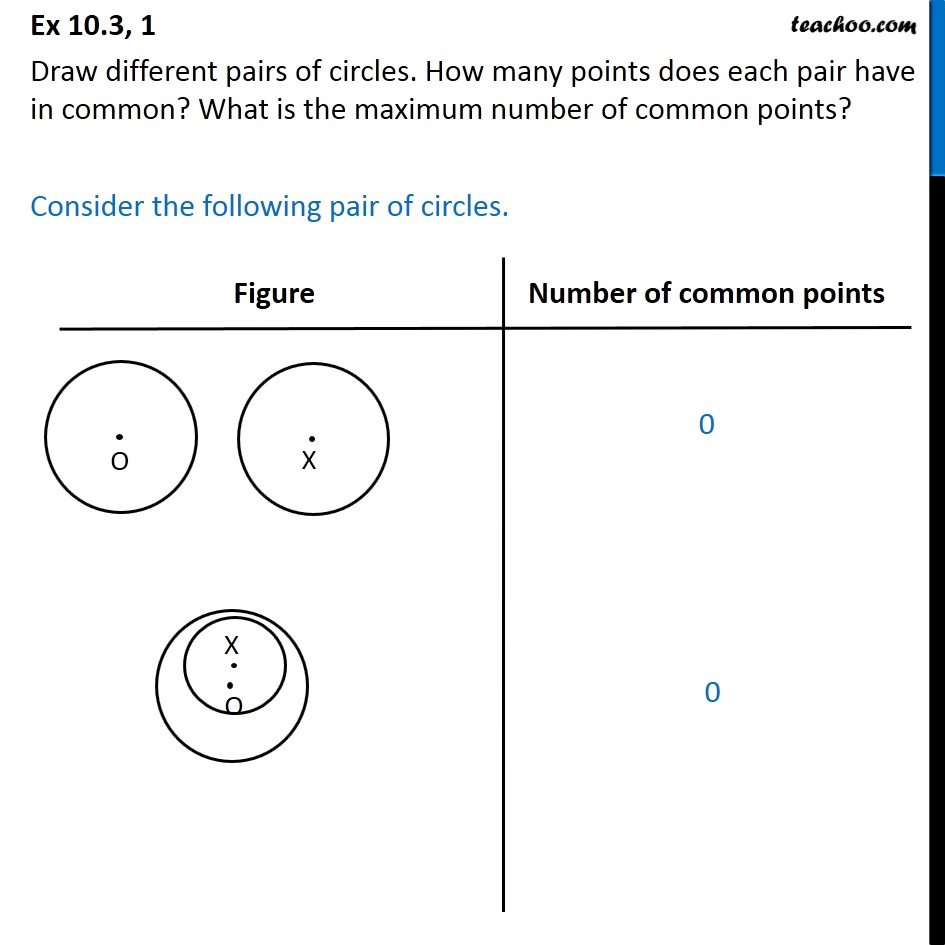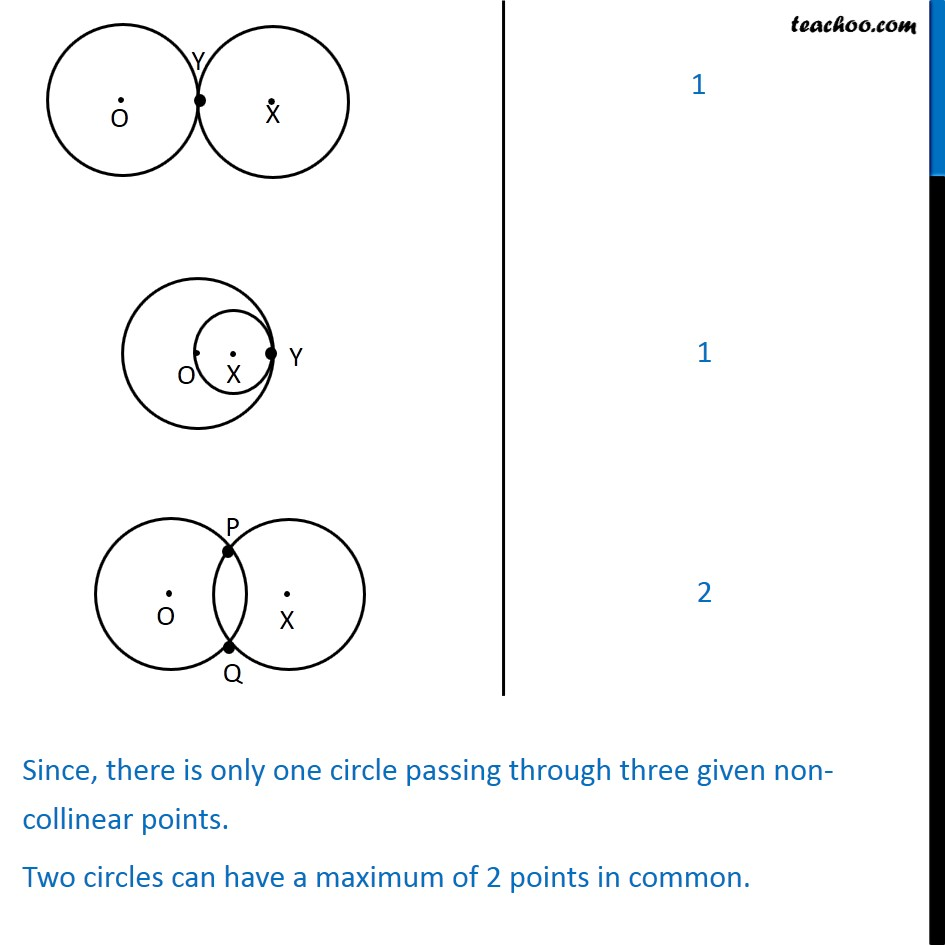1. Chapter 10 Class 9 Circles (Term 2)
2. Serial order wise
3. Ex 10.3

Transcript

Ex 10.3, 1 Draw different pairs of circles. How many points does each pair have in common? What is the maximum number of common points? Consider the following pair of circles. Since, there is only one circle passing through three given non-collinear points. Two circles can have a maximum of 2 points in common.

Ex 10.3